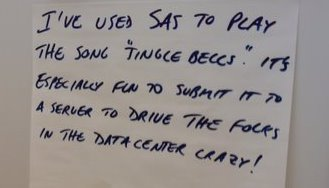It's the holiday season again in the US. Every December, many of us break out the holly, mistletoe, fir and SAS software to ring in the Christmas spirit. What's that? Do you mean to tell me that you don't use the world's leading business analytics software to play Jingle Bells! Well, this user does:Whatever season you are celebrating right now, I hope it isn't crazy.

ShareEditor, Marketing Editorial

+Waynette Tubbs is the Editor of the Risk Management Knowledge Exchange at SAS, Managing Editor of sascom Magazine and Editor of the SAS Tech Report. Tubbs has developed a comprehensive portfolio of strategic business and marketing communications during her career spanning 15 years of magazine, marketing and agency work.

1.Waynette Tubbs on

Colm Smyth (@colmsmyth on Twitter), a SAS user, database designer and Chief blogger for Ireland's Technology Blog, showed me this SAS code for playing Christmas music. He found it on the SAS-L archives..

Date: Fri, 22 Dec 2006 14:46:34 -0500
Subject: Holiday Tune Courtesy of SAS

I'm sure some of you have seen this before, but I figured, I'd send this
out for the holidays.

This was forwarded to me by a colleague of mine who had received it
somewhere else a few years ago.

Copy the code and execute it in SAS.

Merry Christmas and Happy Holidays,

Mark J. Lamias

data holidays;
retain fmtname '@\$holiday';
length data \$3;
ratio = 1.05946309436;
str1 ='A A#B C C#D D#E F F#G G#';
str2='A BbCbB#DbD EbFbE#GbG Ab';
o = 1;
do i = 0 to 87;
p = 55 * ratio**i;
data = compress(substr(str1,mod(i,12)*2+1,2)||o);
output;
if data^=compress(substr(str2,mod(i,12)*2+1,2)||o) then do;
data = compress(substr(str2,mod(i,12)*2+1,2)||o);
output;
end;
if mod(i,12)=2 then o=o+1;
end;
rename data=start p=label;
keep fmtname data p;
run;

proc format cntlin=holidays;
run;

%macro play(input);
data _Null_;
%let i=1;
%do %while(%scan(&input,&i,%str( ))^=);
%let note = %scan(&input,&i,%str( ));
%let pitch = %upcase(%scan(&note,1,=));
%let duration = %scan(&note,2,=);
%let i = %eval(&i+1);
%if &pitch=R %then
call sleep((1/&duration)*750);
%else
call sound(input("&pitch",\$holiday.),(1/&duration)*300);
;
%end;
run;
%mend;

%play(%str(
C6=1 B5=1.5 A5=6 G5=1 R=2 F5=2 E5=1 D5=1 C5=1
R=2 G5=2 A5=1 R=2 A5=2 B5=1 R=2 B5=2 C6=.33
C6=2 C6=2 B5=2 A5=2 G5=2 G5=1.5 F5=4 E5=2
C6=2 C6=2 B5=2 A5=2 G5=2 G5=1.5 F5=4 E5=2 E5=2
E5=2 E5=2 E5=2 E5=4 F5=4 G5=1 R=4 F5=4 E5=4
D5=2 D5=2 D5=2 D5=4 E5=4 F5=1 R=4 E5=4 D5=4
C5=2 C6=1 A5=2 G5=1.5 F5=6 E5=2 F5=2 E5=1 D5=1 C5=1
));

2.Dave Handelsman on

That hand-writing looks awfully familiar.... 🙂

•Has someone been spreading cheer on your server while you weren't looking? Happy holidays, Dave!

3.Wow..

This site uses Akismet to reduce spam. Learn how your comment data is processed.